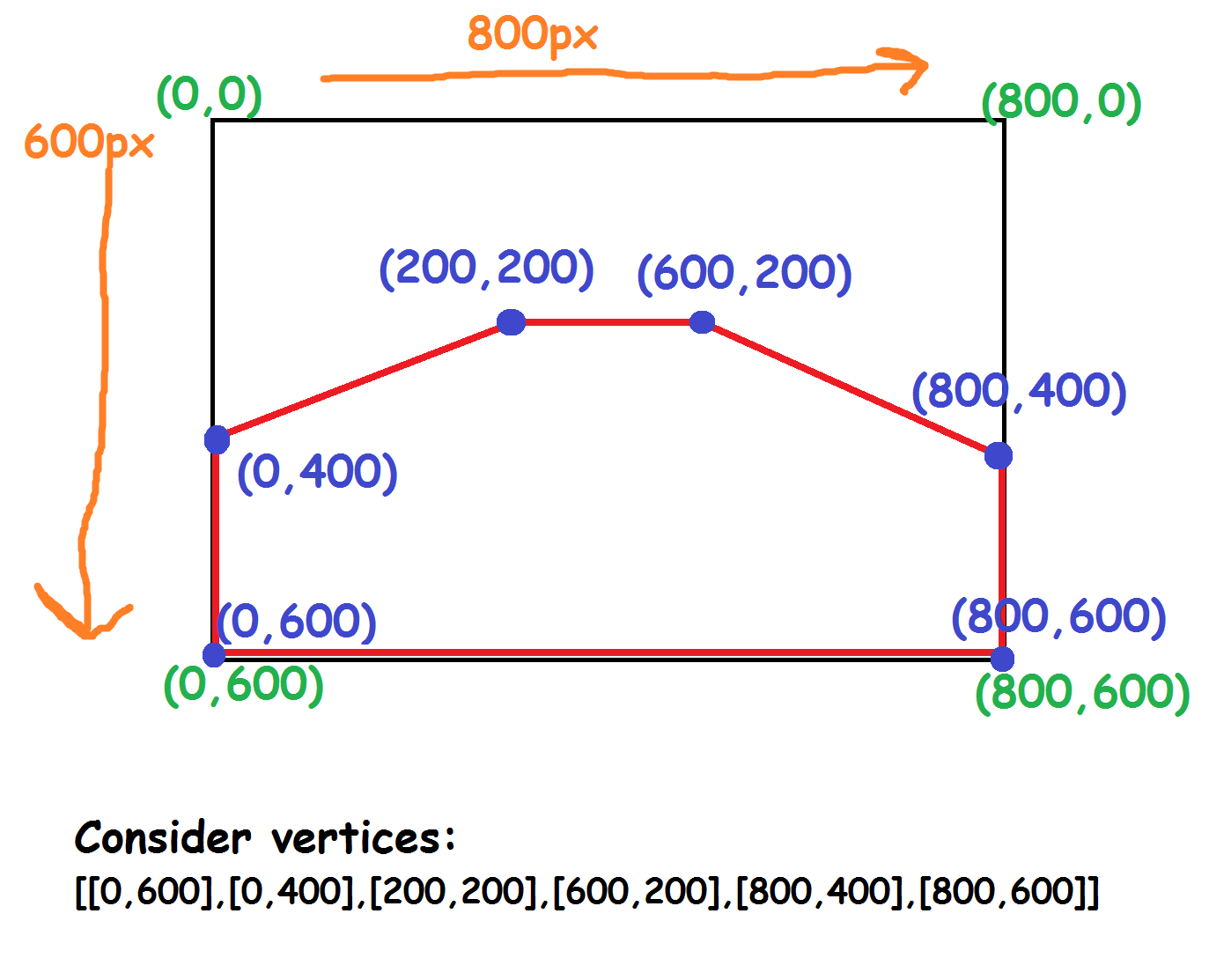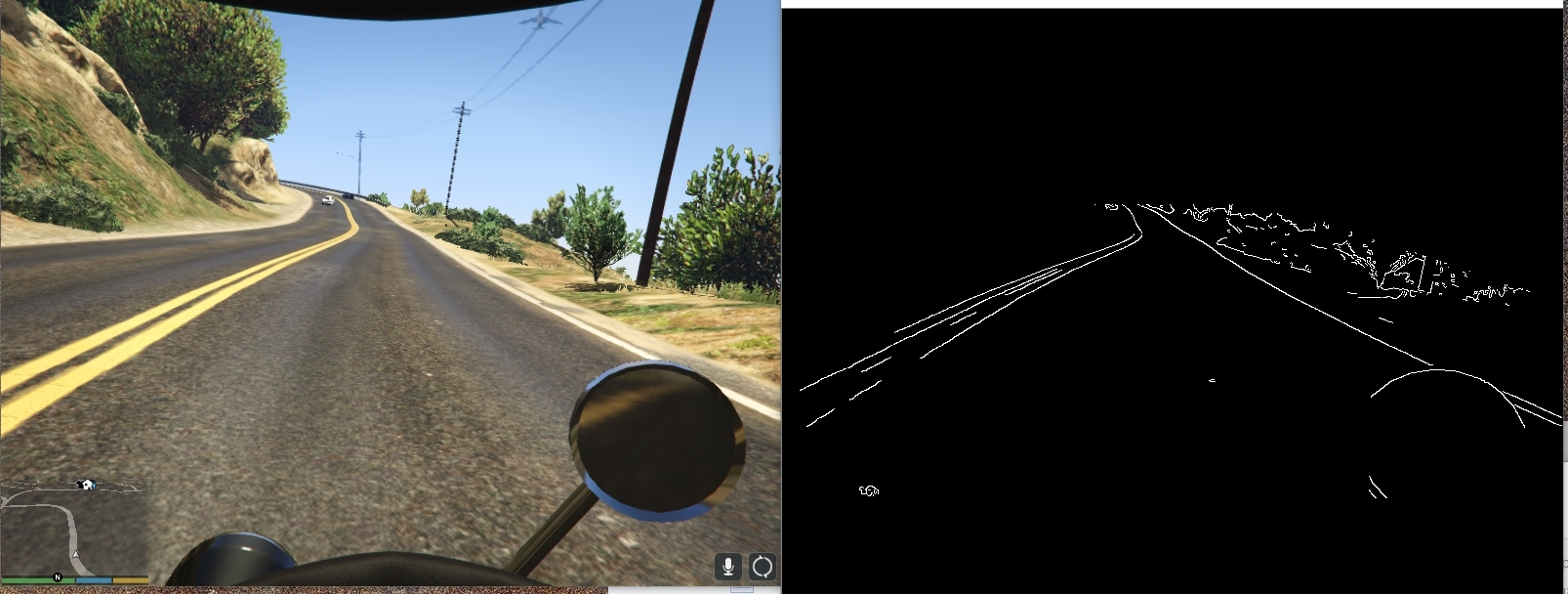## Region of Interest for finding lanes - Python Plays GTA V

pygta5-4-ROI-region-of-interest

Now that we're reading frames, and can do input, we're back on the task of trying to do some self-driving. In order to do this, a common goal is to be able to detect lanes. We can use lane detection to both create a self-driving AI that works based on simple rules based on these lanes and also to train an AI that we hope could later generalize to more scenarios. Code up to this point:

import numpy as np
from PIL import ImageGrab
import cv2
import time
from directkeys import ReleaseKey, PressKey, W, A, S, D

def process_img(original_image):
processed_img = cv2.cvtColor(original_image, cv2.COLOR_BGR2GRAY)
processed_img = cv2.Canny(processed_img, threshold1=200, threshold2=300)
return processed_img

def main():
for i in list(range(4))[::-1]:
print(i+1)
time.sleep(1)

last_time = time.time()
while(True):
screen =  np.array(ImageGrab.grab(bbox=(0,40, 800, 640)))
new_screen = process_img(screen)
print('Loop took {} seconds'.format(time.time()-last_time))
last_time = time.time()
cv2.imshow('window', new_screen)
#cv2.imshow('window2', cv2.cvtColor(screen, cv2.COLOR_BGR2RGB))
if cv2.waitKey(25) & 0xFF == ord('q'):
cv2.destroyAllWindows()
break

def process_img(original_image):
processed_img = cv2.cvtColor(original_image, cv2.COLOR_BGR2GRAY)
processed_img = cv2.Canny(processed_img, threshold1=200, threshold2=300)

vertices = np.array([[10,500],[10,300],[300,200],[500,200],[800,300],[800,500],
], np.int32)
processed_img = roi(processed_img, [vertices])

return processed_img

from IPython.display import Image
Image(filename='idea-of-roi.png')Above is just a quick, not actually scaled right, example of the polygon I will have us using to somewhat illustrate the polygon I've chosen. Now, of course, we're going to need the roi function!

def roi(img, vertices):
# now only show the area that is the mask

def main():
last_time = time.time()
while(True):
screen =  np.array(ImageGrab.grab(bbox=(0,40, 800, 640)))
new_screen = process_img(screen)
print('Loop took {} seconds'.format(time.time()-last_time))
last_time = time.time()
cv2.imshow('window', new_screen)
#cv2.imshow('window2', cv2.cvtColor(screen, cv2.COLOR_BGR2RGB))
if cv2.waitKey(25) & 0xFF == ord('q'):
cv2.destroyAllWindows()
break


Image(filename='roi-and-edge.jpg')As you can see, with this cut down, we wont detect the poles or wires that we otherwise would have in this frame. Next, while we have detected edges, we haven't detected lines. Let's do that!

The next tutorial:• Reading game frames in Python with OpenCV - Python Plays GTA V

• OpenCV basics - Python Plays GTA V

• Direct Input to Game - Python Plays GTA V

• Region of Interest for finding lanes - Python Plays GTA V
• Hough Lines - Python Plays GTA V

• Finding Lanes for our self driving car - Python Plays GTA V

• Self Driving Car - Python Plays GTA V

• Next steps for Deep Learning self driving car - Python Plays GTA V

• Training data for self driving car neural network- Python Plays GTA V

• Balancing neural network training data- Python Plays GTA V

• Training Self-Driving Car neural network- Python Plays GTA V

• Testing self-driving car neural network- Python Plays GTA V

• A more interesting self-driving AI - Python Plays GTA V

• Object detection with Tensorflow - Self Driving Cars in GTA

• Determining other vehicle distances and collision warning - Self Driving Cars in GTA

• Getting the Agent a Vehicle- Python Plays GTA V

• Acquiring a Vehicle for the Agent - Python Plays GTA V